# Read, Analyze and Process SOFA Files

SOFA (Spatially-Oriented Format for Acoustics)  is a file format for storing spatially oriented acoustic data like head-related transfer functions (HRTF) and binaural or spatial room impulse responses. SOFA has been standardized by the Audio Engineering Society (AES) as AES69-2015.

In this example, you load a SOFA file containing HRTF measurements for a single subject in MATLAB. You then analyze the HRTF measurements in the time domain and the frequency domain. Finally, you use the HRTF impulse responses to spatialize an audio signal in real time by modeling a moving source based on desired azimuth and elevation values.

### Load a SOFA File in MATLAB

You use a SOFA file from the SADIE II database . The file corresponds to spatially discrete free-field in-the-ear HRTF measurements for a single subject. The measurements characterize how each ear receives a sound from a point in space.```downloadFolder = matlab.internal.examples.downloadSupportFile("audio","SOFA/SOFA.zip"); dataFolder = tempdir; unzip(downloadFolder,dataFolder) netFolder = fullfile(dataFolder,"SOFA"); addpath(netFolder) filename = "H10_48K_24bit_256tap_FIR_SOFA.sofa";```

#### Display SOFA File Contents

SOFA files consist of binary data stored in the netCDF-4 format. You can use MATLAB to read and write netCDF files.

Display the contents of the SOFA file using `ncdisp` (execute `ncdisp(filename)`).

The file contents consist of multiple fields corresponding to different aspects of the measurements, such as the (fixed) listener position, the varying source position, the coordinate system used to capture the data, general metadata related to the measurement, as well as the measured impulse responses.

NetCDF is a "self-describing" file format, where data is stored along with attributes that can be used to assist in its interpretation. Consider the display snippet corresponding to the source position for example: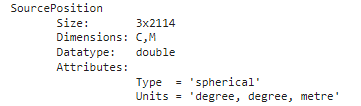`SourcePosition` contains the coordinates for the varying source position used in the measurements (here, there are 2114 separate positions). The file also contains attributes (Type, Units) describing the coordinate system used to store the positions (here, spherical), as well as information about the dimensions of the data (C,M). The dimensions are defined in the AES69 standard :For the file in this example:

• M = 2114 (the total number of measurements, each corresponding to a unique source position).

• R = 2 (corresponding to the two ears).

• E = 1 (one emitter or sound source per measurement).

• N = 256 (the length of each recorded impulse response).

Use `ncinfo` to get information about the SOFA file.

`SOFAInfo = ncinfo(filename);`

The fields of the structure `SOFAInfo` hold information related to the file's dimensions, variables and attributes.

#### Read a Variable from the SOFA File

Use `ncread` to read the value of a variable in the SOFA file.

Read the variable corresponding to the measured impulse responses.

```ir = ncread(filename,"Data.IR"); size(ir)```
```ans = 1×3 256 2 2114 ```

This variable holds impulse responses for the left and right ear for 2114 independent measurements. Each impulse response is of length 256.

Read the sampling rate of the measurements.

`fs = ncread(filename,"Data.SamplingRate")`
```fs = 48000 ```

Plot the first measured impulse response.

```figure; t = (0:size(ir,1)-1)/fs; plot(t,ir(:,1,1)) grid on xlabel("Time (s)") ylabel("Impulse response")```### The SOFA Object

It is possible to read and analyze the contents of the SOFA file using a combination of `ncinfo` and `ncread`. However, the process can be cumbersome and time consuming.

This example introduces a new object, `sofa`, that simplifies this operation. Use `sofa` to read the contents of a SOFA file, analyze the HRTF measurements in the time domain and the frequency domain, and spatialize audio signals based on desired source positions.

Use the `sofa` object to read the contents of the SOFA file.

`s = sofa(filename)`
```s = sofa with properties: IR: [2114×2×256 double] SamplingRate: 48000 SamplingRateUnits: 'hertz' Delay: [0 0] Show all properties ```

Click "Show all properties" in the display above to see the rest of the properties from the SOFA file.

You can easily access the measured impulses responses on the `sofa` object.

```ir = s.IR; size(ir)```
```ans = 1×3 2114 2 256 ```

You access other variables in a similar fashion. For example, read the source positions along with the coordinate system used to express them:

```srcPositions = s.SourcePosition; size(srcPositions)```
```ans = 1×2 2114 3 ```
`srcPositions(1,:)`
```ans = 1×3 0 -90.0000 1.2000 ```
`s.SourcePositionType`
```ans = 'spherical' ```
`s.SourcePositionUnits`
```ans = 'degree, degree, metre' ```

### View the Measurement Geometry

Call `plotGeometry` to view the general geometric setup of the measurements.

```figure; plotGeometry(s) a = gca; a.CameraPosition = [-3 -16 12];```Alternatively, specify input indices to restrict the plot to desired source locations.

```figure; plotGeometry(s,1:100) a = gca; a.CameraPosition = [-3 -16 12];```### Compute HRTF Frequency Responses

The file in this example uses the `SimpleFreeFieldHRIR` convention, which stores impulse response measurements in the time domain as FIR filters.

`s.SOFAConventions`
```ans = 'SimpleFreeFieldHRIR' ```
`s.DataType`
```ans = 'FIR' ```

It is straightforward to compute and plot the frequency response of the impulse responses using `freqz`.

As an illustration, assume you want to plot the frequency response of measurements with an azimuth value in the range of 30 degrees to 32 degrees.

First, inspect the type of the source position data.

`s.SourcePositionType`
```ans = 'spherical' ```
`s.SourcePositionUnits`
```ans = 'degree, degree, metre' ```

The coordinates are spherical, which is convenient for your purpose. Also, note that the units are in degrees, so conversion from radians per second to degrees in not necessary.

Per the SOFA standard , the first angle value corresponds to the azimuth.

`az = s.SourcePosition(:,1);`

Find the values corresponding to the desired azimuth range.

`indices = find(az>30 & az<32);`

You are interested in the impulse responses corresponding to the first receiver (ear) only. Read the corresponding impulse responses.

```receiver = 1; IR = s.IR(indices,receiver,:); IR = permute(IR,[3 1 2]); IR = squeeze(IR); size(IR)```
```ans = 1×2 256 22 ```

Use `freqz` to compute the frequency response of each FIR filter. Specify a 4096-point frequency response.

```N = 4096; H = zeros(N,length(indices)); for index = 1:length(indices) [H(:,index),F] = freqz(IR(:,index),1, N ,s.SamplingRate); end```

Plot the magnitude responses.

```figure for index=1:length(indices) plot(F,20*log10(abs(H(:,index)))); hold on end grid on ylabel("Magnitude (dB)"); xlabel("Frequency (Hz)")```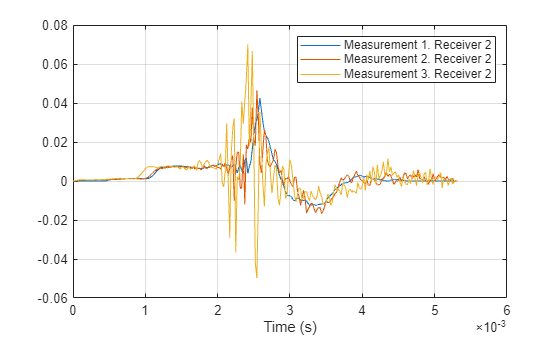You can also accomplish this task by calling `freqz` on the `sofa` object directly. As an example, plot the frequency responses of the first 5 impulse responses of the second receiver.

```figure freqz(s,Receiver=2,Indices=1:5)```### Compute HRTF Spectra

It is often useful to compute and visualize the magnitude spectra of HRTF data in a specific plane in space.

For example, compute the spectrum in the horizontal plane (corresponding to an elevation angle equal to zero) for the first receiver.

Find measurements with an elevation angle within 2 degrees of zero.

```threshold = 2; ele = s.SourcePosition(:,2); indices = find(abs(ele)<threshold); ```

Read the corresponding impulse responses for one ear.

```receiver = 1; IR = s.IR(indices,receiver,:); IR = permute(IR,[3 1 2]); IR = squeeze(IR); size(IR)```
```ans = 1×2 256 96 ```

Use `freqz` to compute the frequency response of each FIR filter. Specify a 4096-point frequency response.

```N = 4096; H = zeros(N,length(indices)); for index = 1:length(indices) [H(:,index),F] = freqz(IR(:,index),1, N ,s.SamplingRate); end```

Convert to log scale.

`H = 20*log10(abs(H)).';`

Eliminate small values by using a noise floor.

```noiseFloor = -50; H(H<noiseFloor) = noiseFloor;```

Sort data by azimuth values. Note that the SOFA standard uses an azimuth range of [0, 360] degrees. Convert it to [-180,180] degrees.

```azi = s.SourcePosition(indices,1); azi(azi>180)=azi(azi>180)-360; [azi,ind]=sort(azi); H = H(ind,:);```

Plot the horizontal plane spectrum.

```figure surface(F.',azi,H(:,:)); shading flat xlabel("Frequency (Hz)"); ylabel("Azimuth (deg)"); colorbar; axis tight```You can also plot the horizontal spectrum by directly using the `spectrum` method of the `sofa` object.

Use the spectrum method to plot the spectrum in the horizontal plane for the second receiver.

```figure; spectrum(s, Receiver=2)```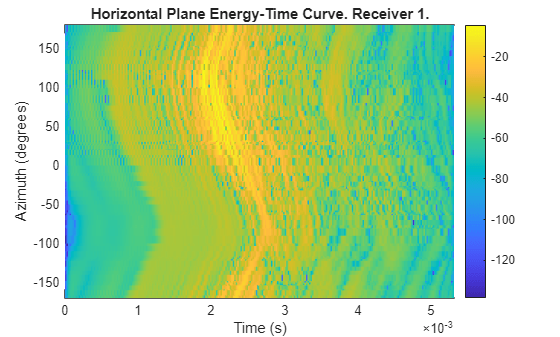### Compute Energy-Time Curve

It is often useful to visualize the decay of the HRTF responses over time using an energy-time curve (ETC).

In this section, you measure and plot the ETC in the horizontal plane. You use the same impulse responses you used to compute the magnitude spectrum in the horizontal plane in the previous section.

Convert the impulse response values to the log domain.

`IRLog = 20*log10(abs(IR));`

Similar to the previous section, sort the data by azimuth values.

`IRLog = IRLog(:,ind);`

Eliminate values smaller than the noise floor.

```noiseFloor=-50; IRLog(IRLog<=noiseFloor)=noiseFloor;```

Plot the ETC.

```fs = s.SamplingRate; t = 0:1/fs*1000:(size(IRLog,1)-1)/fs*1000; figure surface(t,azi,IRLog.'); set(gca,FontName="Arial",FontSize=10); set(gca, TickLength=[0.02 0.05]); set(gca,LineWidth=1); cmap=colormap(hot); cmap=flipud(cmap); shading flat colormap(cmap); box on; colorbar; xlabel("Time (ms)"); ylabel("Azimuth (deg)"); axis tight grid on```Use the `energyTimeCurve` method of the `sofa` object to generate such ETC visualizations. For example, visualize the ETC in the horizontal plane for the second receiver.

```figure; energyTimeCurve(s, Receiver=2)```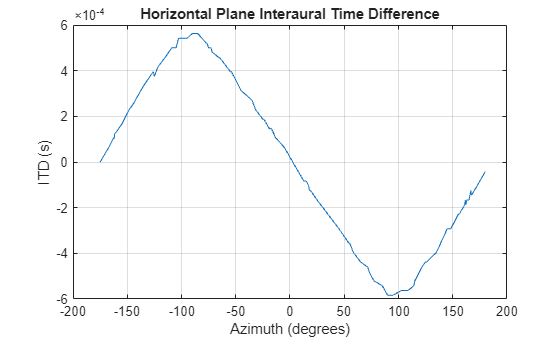### Compute Interaural Time Delay

Interaural time delay (ITD) is the difference in arrival time of a sound between two ears. It is an important binaural cue for sound source localization. There are many ITD estimation methods (see ).Here, you compute the ITD using a simple cross-correlation technique.

First, pass the impulse responses through a lowpass filter.

Design the lowpass filter.

```cutOffFreq = 3000; fs = s.SamplingRate; cutOffFreqNorm = cutOffFreq/(fs/2); [b,a] = butter(5,cutOffFreqNorm);```

Filter the impulse responses.

`ir = filter(b,a,s.IR,[],3);`

Estimate the delay between the left and right ear responses using a cross-correlation metric.

```pos = size(ir,1); toa_diff = zeros(1,pos); N = size(ir,3); for ii=1:pos cc = xcorr(squeeze(ir(ii,1,:)),squeeze(ir(ii,2,:))); [~,idx_lag] = max(abs(cc)); toa_diff(ii) = idx_lag - N; end toa_diff = toa_diff/fs;```

You can perform the same task by calling the `interauralTimeDelay `method of the `sofa` object. To get a utility plot displaying ITD for all measurements, call the method with no output arguments.

`interauralTimeDelay(s)`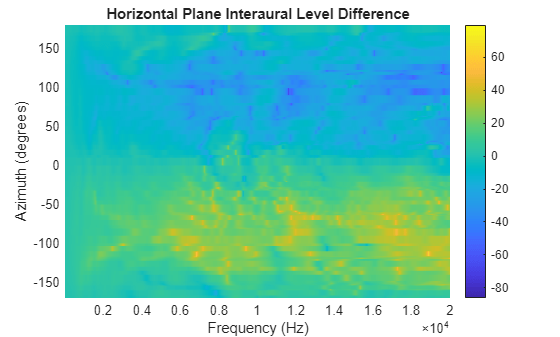Plot ITD versus the azimuth angle in the horizontal plane using the method `horizontalITD`. The method leverages `polarplot`.

`horizontalITD(s);`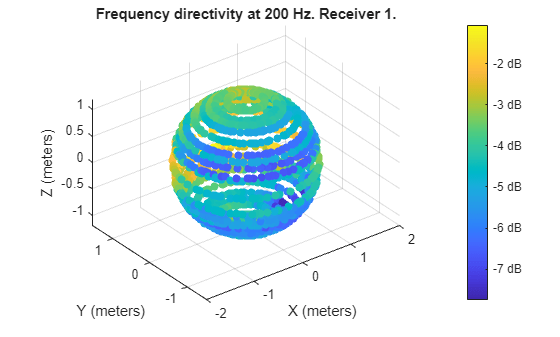### Interpolate HRTF Measurements

The HRTF measurements in the SOFA file correspond to a finite number of azimuth/elevation angle combinations. It is possible to interpolate the data to any desired spatial location using 3-D HRTF interpolation with `interpolateHRTF`.

Specify the desired source position (in degrees).

```desiredAz = [-120;-60;0;60;120;0;-120;120]; desiredEl = [-90;90;45;0;-45;0;45;45]; desiredPosition = [desiredAz, desiredEl];```

Read the measured source positions stored in the SOFA file.

```pos = s.SourcePosition; sourcePosition = pos(:,1:2);```

Per the SOFA standard, azimuth values are stored in the range zero to 360 degrees. Since the desired azimuth values contain negative angles, switch the stored values to the range -180 to 180 degrees.

```azi = sourcePosition(:,1); azi=mod(azi,360); idx=find(azi>180 & azi<=360); azi(idx) = -(360-azi(idx)); sourcePosition(:,1) = azi;```

`hrtfData = s.IR;`

Calculate the head-related impulse response (HRIR) using the vector base amplitude panning interpolation (VBAP) algorithm at a desired source position.

```interpolatedIR = interpolateHRTF(hrtfData, ... sourcePosition, ... desiredPosition);```

The above steps break down how to use `interpolateHRTF` with the SOFA data. You can accomplish this task easily by directly calling the `interpolateHRTF` method of the `sofa` object:

`interpolatedIR2 = interpolateHRTF(s,desiredPosition);`

Separate the output into the impulse responses of the left and right ears.

```leftIR = squeeze(interpolatedIR(:,1,:)); rightIR = squeeze(interpolatedIR(:,2,:));```

#### Model Moving Source Using HRIR Filtering

Filter a mono input through the interpolated impulse responses to model a moving source.

Create an audio file sampled at 48 kHz for compatibility with the HRTF dataset.

```desiredFs = s.SamplingRate; [audio,fs] = audioread("Counting-16-44p1-mono-15secs.wav"); audiowrite("Counting-16-48-mono-15secs.wav",audio,desiredFs);```

Create a `dsp.AudioFileReader` object to read in a file frame by frame. Create an `audioDeviceWriter` object to play audio to your sound card frame by frame. Create two `dsp.FIRFilter` objects with `NumeratorSource` set to `Input port`. Setting `NumeratorSource` to `Input port` enables you to modify the filter coefficients while streaming.

```fileReader = dsp.AudioFileReader("Counting-16-48-mono-15secs.wav"); deviceWriter = audioDeviceWriter(SampleRate=fileReader.SampleRate); leftFilter = dsp.FIRFilter(NumeratorSource="Input port"); rightFilter = dsp.FIRFilter(NumeratorSource="Input port");```

In an audio stream loop:

1. Read in a frame of audio data.

2. Feed the audio data through the left and right HRIR filters.

3. Concatenate the left and right channels and write the audio to your output device. If you have a stereo output hardware, such as headphones, you can hear the source shifting position over time.

4. Modify the desired source position in 2-second intervals by updating the filter coefficients.

```durationPerPosition = 2; samplesPerPosition = durationPerPosition*fileReader.SampleRate; samplesPerPosition = samplesPerPosition - rem(samplesPerPosition,fileReader.SamplesPerFrame); sourcePositionIndex = 1; samplesRead = 0; while ~isDone(fileReader) audioIn = fileReader(); samplesRead = samplesRead + fileReader.SamplesPerFrame; leftChannel = leftFilter(audioIn,leftIR(sourcePositionIndex,:)); rightChannel = rightFilter(audioIn,rightIR(sourcePositionIndex,:)); deviceWriter([leftChannel,rightChannel]); if mod(samplesRead,samplesPerPosition) == 0 sourcePositionIndex = sourcePositionIndex + 1; end end```

As a best practice, release your System objects when complete.

```release(deviceWriter) release(fileReader)```

### Spatialize Audio in Real Time

You can use the `sofa` object directly to simulate a sound source moving in space.

Simulate a sound source moving in the horizontal plane, with an initial azimuth of -90 degrees. Gradually increase the azimuth in the loop. Pass the audio frames to the `sofa` object along with the desired source location.

```index = 1; loc = [-90 0]; while ~isDone(fileReader) index=index+1; frame = fileReader(); frame = frame(:,1); y = s(frame,loc); deviceWriter(y); if mod(index,100)==0 loc(1)=loc(1)+30; end end```
```release(deviceWriter) release(fileReader)```

### References

 SOFA (Spatially Oriented Format for Acoustics). https://www.sofaconventions.org

 AES69-2022: AES standard for file exchange - Spatial acoustic data file format

 Andreopoulou A, Katz BFG. Identification of perceptually relevant methods of inter-aural time difference estimation. J Acoust Soc Am. 2017 Aug;142(2):588. doi: 10.1121/1.4996457. PMID: 28863557.